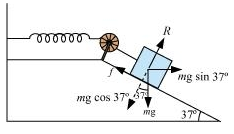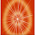## Pages

### Work, Energy and Power NCERT Solutions Class 11 Physics - Solved Exercise Question 6.26

Question 6.26:
A 1 kg block situated on a rough incline is connected to a spring of spring constant 100 N m–1 as shown in Fig. 6.17. The block is released from rest with the spring in the unstretched position. The block moves 10 cm down the incline before coming to rest. Find the coefficient of friction between the block and the incline. Assume that the spring has a negligible mass and the pulley is frictionless.Mass of the block, m = 1 kg
Spring constant, k = 100 N m–1
Displacement in the block, x = 10 cm = 0.1 m
The given situation can be shown as in the following figure.At equilibrium:
Normal reaction, R = mg cos 37°
Frictional force, f = μ R = mg Sin 370
Where, μ is the coefficient of friction
Net force acting on the block = mg sin 37° – f
= mgsin 37° – μmgcos 37°
= mg(sin 37° – μcos 37°)
At equilibrium, the work done by the block is equal to the potential energy of the spring, i.e.,
mg(sin 37° – μcos 37°)x = (1/2)kx2
1 X 9.8 (Sin 370 - μcos 37°) = (1/2) X 100 X (0.1)
0.602 - μ X 0.799 = 0.510
∴ μ = 0.092 / 0.799  =  0.115

1.2.